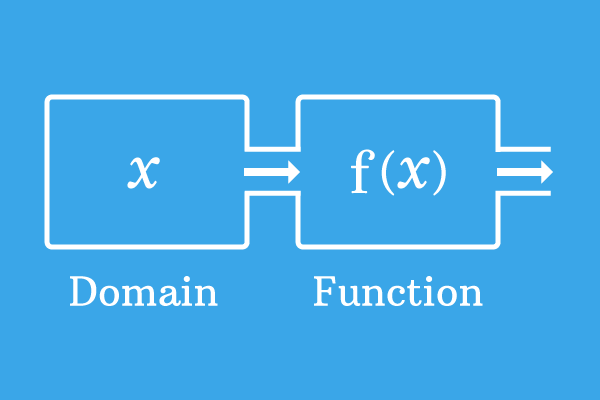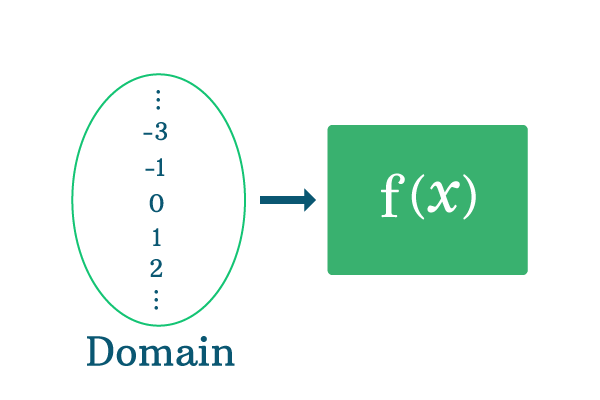DomainA set of elements for which a function has been defined is called the domain of a function.

Every function is defined for some elements by giving their associated values. The collection of such elements as a group is known as domain of the function.

Each function has a domain and it helps us to understand the list of values for which a function is defined mathematically.

Introduction

Consider a function and assume it is defined for the elements $a$, $b$, $c$, $d$ and $e$. Take $x$ as a literal and it represents all these elements. Assume, the function is expressed as $f(x)$ in mathematics.Take $x = a$, then the value of the function is $f(a)$

Take $x = b$, then the value of the function is $f(b)$

Take $x = c$, then the value of the function is $f(c)$

Take $x = d$, then the value of the function is $f(d)$

Take $x = e$, then the value of the function is $f(e)$

The values of function are $f(a)$, $f(b)$, $f(c)$, $f(d)$ and $f(e)$ for $x$ is equal to $a$, $b$, $c$, $d$ and $e$ respectively. The collection of the elements $a$, $b$, $c$, $d$ and $e$ is called the domain of the function.

$x = \{a, b, c, d, e\}$

Example

$f(x) = \dfrac{1}{x+2}$

Substitute all the real numbers to determine the domain of this function.

For example, Take $x = 0$ and evaluate the function.

$x = 0$ $\implies$ $f(0) = \dfrac{1}{0+2} = \dfrac{1}{2}$

For $x$ is equal to zero, the value of the function is $\dfrac{1}{2}$. Now, substitute positive real numbers and obtain their associated values.

$x = 1$ $\implies$ $f(1) = \dfrac{1}{1+2} = \dfrac{1}{3}$

$x = 2$ $\implies$ $f(2) = \dfrac{1}{2+2} = \dfrac{1}{4}$

$x = 3$ $\implies$ $f(3) = \dfrac{1}{3+2} = \dfrac{1}{5}$

$x = 4$ $\implies$ $f(4) = \dfrac{1}{4+2} = \dfrac{1}{6}$

$x = 5$ $\implies$ $f(5) = \dfrac{1}{5+2} = \dfrac{1}{7}$

$\vdots$

The function is defined for all the positive real numbers.

Now, substitute all the negative real numbers one by one and test the functionality of this function.

$x = -1$ $\implies$ $f(-1) = \dfrac{1}{-1+2} = \dfrac{1}{1} = 1$

$x = -2$ $\implies$ $f(-2) = \dfrac{1}{-2+2} = \dfrac{1}{0} = \infty$

For the value $x$ is equal to $-2$, the example function becomes undefined. Hence, the value of $x$ should not be equal to $-2$. Continue testing the function by substituting the remaining negative real numbers.

$x = -3$ $\implies$ $f(-3) = \dfrac{1}{-3+2} = \dfrac{1}{-1} = -1$

$x = -4$ $\implies$ $f(-4) = \dfrac{1}{-4+2} = \dfrac{1}{-2} = -\dfrac{1}{2}$

$x = -5$ $\implies$ $f(-5) = \dfrac{1}{-5+2} = \dfrac{1}{-3} = -\dfrac{1}{3}$

$\vdots$Now, collect all the elements as a set but ignore $x$ is equal to $-2$. Therefore, the collection of elements is called the domain of this function.

$x = \{\cdots -4, -3, -1, 0, 1, 2, 3, \cdots\}$

According to the set theory, the real numbers group is simplify denoted by $R$ but it contains $-2$. Hence, it should be subtracted from it. Therefore, the domain of the function is simplify written as $R-\{0\}$.

Latest Math Topics
Latest Math Problems
Email subscription
Math Doubts is a best place to learn mathematics and from basics to advanced scientific level for students, teachers and researchers. Know more